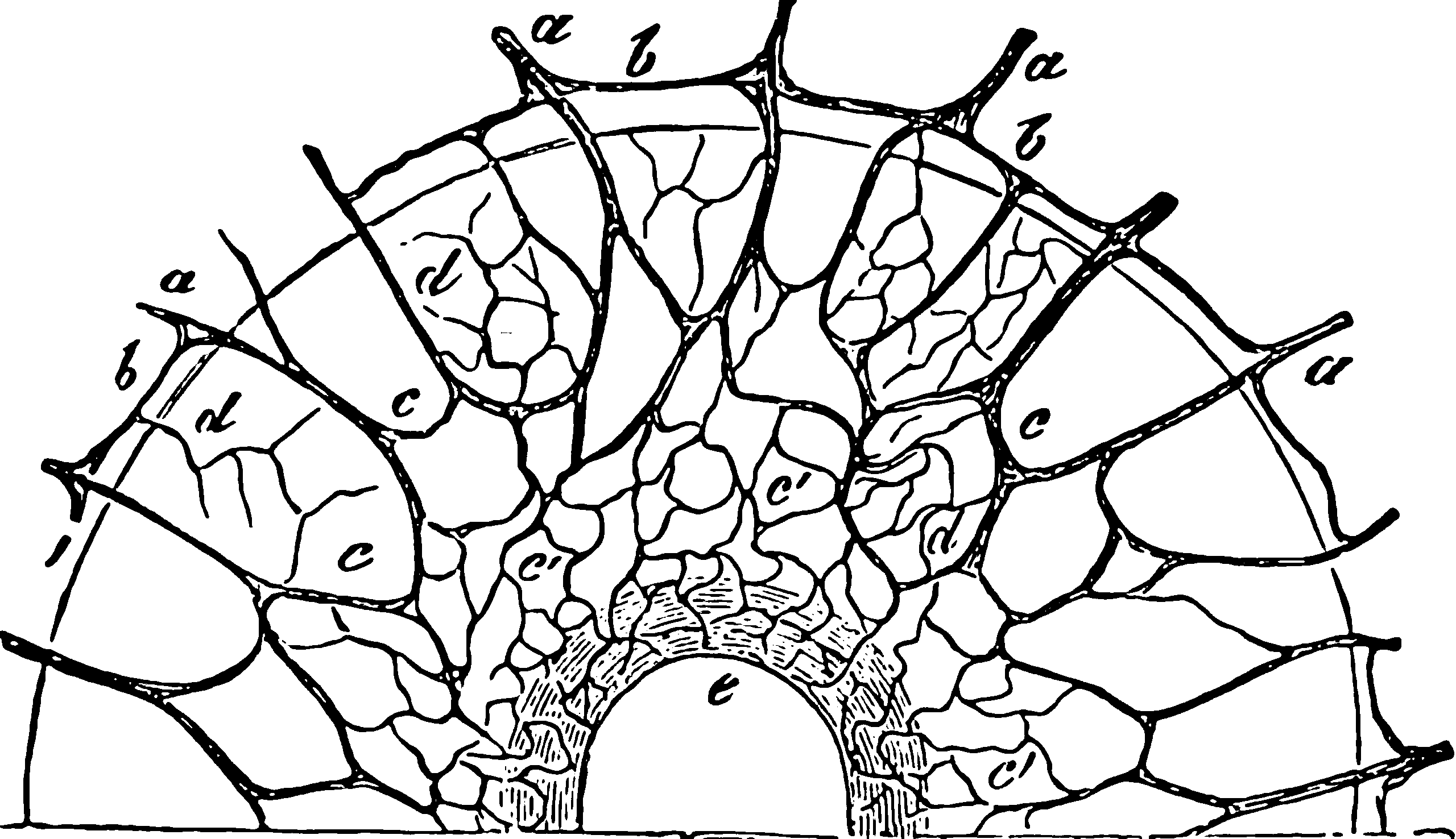# Graph neural netsNeural networks applied to graph data. Neural networks of course can already be represented as directed graphs, or applied to phenomena which arise from a causal graph but that is not what we mean here. What we mean here is using information about graph topology as a feature input (and possibly output) for a neural network. In practice this is usually some variant of applying convnets to spectral graph representations; there is an obvious analogy to generic graph computations and belief propagation but I do not know how those play our in practice.

I am not closely following this area at the moment, so be aware content may not be current.

## Tutorials

Pantelis Elinas wrote a good tutorial. One of his motivating examples is nifty: He argues graph learning is powerful because it includes the fundamental problem of discovering knowledge graphs and thus research discovery. He recommends the following summaries: Bronstein et al. (2017);Bronstein et al. (2021);Hamilton (2020);Hamilton, Ying, and Leskovec (2018);Xia et al. (2021).

Chami et al. (2022):

There has been a surge of recent interest in graph representation learning (GRL). GRL methods have generally fallen into three main categories, based on the availability of labeled data. The first, network embedding, focuses on learning unsupervised representations of relational structure. The second, graph regularized neural networks, leverages graphs to augment neural network losses with a regularization objective for semi-supervised learning. The third, graph neural networks, aims to learn differentiable functions over discrete topologies with arbitrary structure. However, despite the popularity of these areas there has been surprisingly little work on unifying the three paradigms. Here, we aim to bridge the gap between network embedding, graph regularization and graph neural networks. We propose a comprehensive taxonomy of GRL methods, aiming to unify several disparate bodies of work. Specifically, we propose the GraphEDM framework, which generalizes popular algorithms for semi-supervised learning (e.g. GraphSage, GCN, GAT), and unsupervised learning (e.g. DeepWalk, node2vec) of graph representations into a single consistent approach. To illustrate the generality of GraphEDM, we fit over thirty existing methods into this framework. We believe that this unifying view both provides a solid foundation for understanding the intuition behind these methods, and enables future research in the area.

## Fun tweaks

Distance Encoding is a general class of graph-structure-related features that can be utilized by graph neural networks to improve the structural representation power. Given a node set whose structural representation is to be learnt, DE for a node over the graph is defined as a mapping of a set of landing probabilities of random walks from each node of the node set of interest to this node. Distance encoding generally includes measures such as shortest-path-distances and generalized PageRank scores. Distance encoding can be merged into the design of graph neural networks in simple but effective ways: First, we propose DEGNN that utilizes distance encoding as extra node features. We further enhance DEGNN by allowing distance encoding to control the aggregation procedure of traditional GNNs, which yields another model DEAGNN. Since distance encoding purely depends on the graph structure and is independent from node identifiers, it has inductive and generalization ability.

## pytorch geometric

### deep graph library

Build your models with PyTorch, TensorFlow or Apache MXNet.

Fast and memory-efficient message passing primitives for training Graph Neural Networks. Scale to giant graphs via multi-GPU acceleration and distributed training infrastructure.

### GTN

GTN is an open source framework for automatic differentiation with a powerful, expressive type of graph called weighted finite-state transducers (WFSTs). ust as PyTorch provides a framework for automatic differentiation with tensors, GTN provides such a framework for WFSTs. AI researchers and engineers can use GTN to more effectively train graph-based machine learning models.

I have not used GTN so I cannot say if I have filed this item correctly or if it is more of a computational graph learning tool.

## Background: Graph filtering

A lot of this seems to be based upon more classic linear systems theory applied to networks in the form of spectral graph theory. See signal processing on graphs.

## Connection to graphical model learning

e.g. Yu et al. (2019).

### No comments yet. Why not leave one?

GitHub-flavored Markdown & a sane subset of HTML is supported.